# Civil Engineering - UPSC Civil Service Exam Questions

16.

Total elongation of a prismatic bar of length L, weight W, cross-sectional area A and modulus of elasticity E, under its own weight, while hanging vertically, is

 A.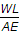B.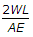C.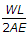D.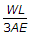Explanation:

No answer description available for this question. Let us discuss.

17.

For a partially saturated soil, Δu the increase in pore water pressure, when no drainage is permitted, is expressed as (where A and B are Skempton's pore pressure parameters and Δσ1 and Δσ3 are major and minor principal stress increments)

 A. Δu = B[Δσ3 + A(Δσ1 - Δσ3)] B. Δu = A[Δσ3 + B(Δσ1 - Δσ3)] C. Δu = Δσ3 + A(Δσ1 - Δσ3)] D. Δu = Δσ3 + B(Δσ1 - Δσ3)]

Explanation:

No answer description available for this question. Let us discuss.

18.

The shear force diagram for a simple supported beam of span 'l' is given in the figure. The maximum bending moment is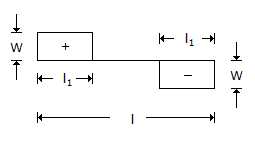A.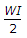B.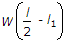C. Wl1 D. W(l - 2l1)

Explanation:

No answer description available for this question. Let us discuss.

19.

A compound bar consisting of material A and B is tightly secured at the ends. The coefficients of thermal expansion of A is more than that of B. When the temperature is increased the stresses induced will be

 A. tensile in both the materials B. tensile in material A and compressive in material B C. compressive in material A and tensile in material B D. compressive in both the materials

Explanation:

No answer description available for this question. Let us discuss.

20.

Couple M is applied at C on a simply supported beam AB. What is the maximum shear force for the beam ?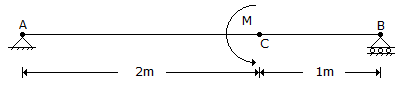A. Zero B. M C. 2 M/3 D. M/3